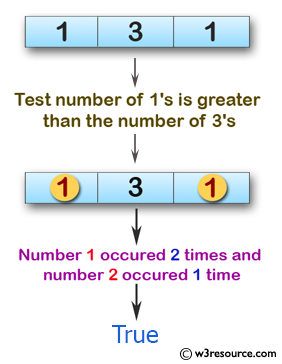﻿ Swift Array Programming Exercise: Test if the number of 1's is greater than the number of 3's of a given array of integers - w3resource# Swift Array Programming Exercises: Test if the number of 1's is greater than the number of 3's of a given array of integers

## Swift Array Programming: Exercise-28 with Solution

Write a Swift program to test if the number of 1's is greater than the number of 3's of a given array of integers.

Pictorial Presentation:Sample Solution:

Swift Code:

``````func test_nums(array_nums: [Int]) -> Bool {
var p = 0
var q = 0

for x in 0..<array_nums.count {
if array_nums[x] == 1 {
p += 1
}
else if array_nums[x] == 3 {
q += 1
}
}

return p > q
}

print(test_nums(array_nums: [1, 3, 1]))
print(test_nums(array_nums: [1, 3, 1, 3, 2]))
print(test_nums(array_nums: [3, 3]))
```
```

Sample Output:

```true
false
false
```

Swift Programming Code Editor:

Improve this sample solution and post your code through Disqus

What is the difficulty level of this exercise?

﻿